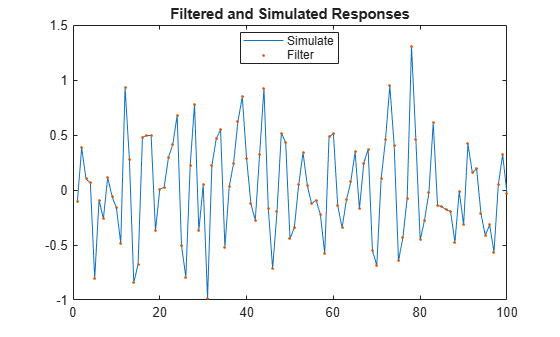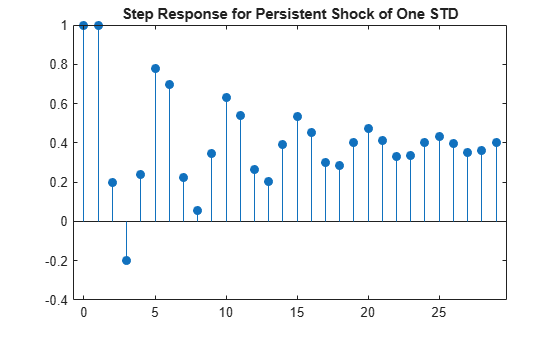# filter

Class: regARIMA

Filter disturbances through regression model with ARIMA errors

## Syntax

[Y,E,U] = filter(Mdl,Z) [Y,E,U] = filter(Mdl,Z,Name,Value) 

## Description

[Y,E,U] = filter(Mdl,Z) filters errors to produce responses, innovations, and unconditional disturbances of a univariate regression model with ARIMA time series errors.

[Y,E,U] = filter(Mdl,Z,Name,Value) filters errors using additional options specified by one or more Name,Value pair arguments.

## Input Arguments

 Mdl Regression model with ARIMA errors, specified as a model returned by regARIMA or estimate. The parameters of Mdl cannot contain NaNs. Z Errors that drive the innovation process, specified as a numObs-by-numPaths matrix. That is, εt = σzt is the innovations process, where σ is the innovation standard deviation and zt are the errors for t = 1,...,T. As a column vector, Z represents a path of the underlying error series. As a matrix, Z represents numObs observations of numPaths paths of the underlying errors. filter assumes that observations across any row occur simultaneously. The last row contains the latest observation. Z is a continuation of the presample errors, Z0.

### Name-Value Arguments

Specify optional pairs of arguments as Name1=Value1,...,NameN=ValueN, where Name is the argument name and Value is the corresponding value. Name-value arguments must appear after other arguments, but the order of the pairs does not matter.

Before R2021a, use commas to separate each name and value, and enclose Name in quotes.

 U0 Presample unconditional disturbances that provide initial values for the ARIMA error model, specified as the comma-separated pair consisting of 'U0' and a column vector or matrix. If U0 is a column vector, then filter applies it to each output path.If U0 is a matrix, then it requires at least numPaths columns. If the number of columns exceeds numPaths, then filter uses the first numPaths columns.U0 requires enough rows to initialize the compound autoregressive component of the ARIMA error model. The required number of rows is at least Mdl.P. If the number of rows in U0 exceeds the number necessary, then filter uses the latest Mdl.P presample unconditional disturbances. The last row contains the latest presample unconditional disturbance. Default: filter sets the necessary presample unconditional disturbances to 0. X Predictor data in the regression model, specified as the comma-separated pair consisting of 'X' and a matrix. The columns of X are separate, synchronized time series, with the last row containing the latest observations. The number of rows of X must be at least numObs. If the number of rows of X exceeds the number necessary, then filter uses the latest observations. Default: filter does not include a regression component in the model regardless of the presence of regression coefficients in Mdl. Z0 Presample errors providing initial values for the input error series, Z, specified as the comma-separated pair consisting of 'Z0' and a vector or matrix. If Z0 is a column vector, then filter applies it to each output path. If Z0 is a matrix, then it requires at least numPaths columns. If the number of columns exceeds numPaths, then filter uses the first numPaths columns.Z0 requires enough rows to initialize the compound moving average component of the ARIMA error model. The required number of rows is at least Mdl.Q. If the number of rows in Z0 exceeds the number necessary, then filter uses the latest Mdl.Q observations. The last row contains the latest observation. Default: filter sets the necessary presample errors to 0.

Notes

• NaNs in Z, U0, X, and Z0 indicate missing values and filter removes them. The software merges the presample data sets (U0 and Z0), then uses list-wise deletion to remove any NaNs. filter similarly removes NaNs from the effective sample data (Z and X). Removing NaNs in the data reduces the sample size. Such removal can also create irregular time series.

• Removing NaNs in the main data reduces the effective sample size. Such removal can also create irregular time series.

• filter assumes that you synchronize presample data such that the latest observation of each presample series occurs simultaneously.

• All predictor series (i.e. columns) in X are associated with each error series in Z to produce numPaths response series Y.

## Output Arguments

 Y Simulated responses, returned as a numobs-by-numPaths matrix. E Simulated, mean 0 innovations of the ARIMA error model, returned as a numobs-by-numPaths matrix. U Simulated unconditional disturbances, returned as a numobs-by-numPaths matrix.

## Examples

expand all

Simulate responses using filter and simulate. Then compare the simulated responses.

Both filter and simulate filter a series of errors to produce output responses y, innovations e, and unconditional disturbances u. The difference is that simulate generates errors from Mdl.Distribution, whereas filter accepts a random array of errors that you generate from any distribution.

Specify the following regression model with ARMA(2,1) errors:

$\begin{array}{l}\begin{array}{c}{y}_{t}={X}_{t}\left[\begin{array}{c}0.1\\ -0.2\end{array}\right]+{u}_{t}\\ {u}_{t}=0.5{u}_{t-1}-0.8{u}_{t-2}+{\epsilon }_{t}-0.5{\epsilon }_{t-1},\end{array}\end{array}$

where ${\epsilon }_{t}$ is Gaussian with variance 0.1.

Mdl = regARIMA('Intercept',0,'AR',{0.5 -0.8}, ... 'MA',-0.5,'Beta',[0.1 -0.2],'Variance',0.1);

Simulate data for the predictors and from Mdl using Monte Carlo simulation.

rng(1); % For reproducibility X = randn(100,2); % Simulate predictor data [ySim,eSim,uSim] = simulate(Mdl,100,'X',X);

Standardize the simulated innovations and filter them.

z1 = eSim./sqrt(Mdl.Variance); [yFlt1,eFlt1,uFlt1] = filter(Mdl,z1,'X',X);

Confirm that the simulated responses from simulate and filter are identical using a plot.

figure h1 = plot(ySim); hold on h2 = plot(yFlt1,'.'); title '{\bf Filtered and Simulated Responses}'; legend([h1, h2],'Simulate','Filter','Location','Best') hold offAlternatively, simulate responses by randomly generating your own errors and passing them into filter.

rng(1); X = randn(100,2); z2 = randn(100,1); yFlt2 = filter(Mdl,z2,'X',X); figure h1 = plot(ySim); hold on h2 = plot(yFlt2,'.'); title '{\bf Filtered and Simulated Responses}'; legend([h1, h2],'Simulate','Filter','Location','Best') hold offThis plot is the same as the previous plot, confirming that both simulation methods are equivalent.

filter multiplies the error, Z, by sqrt(Mdl.Variance) before filtering Z through the model. Therefore, if you want to specify your own distribution, set Mdl.Variance to 1, and then generate your own errors using, for example, random('unif',a,b) for the Uniform(a, b) distribution.

Simulate the impulse response of an innovation shock to the regression model with ARMA(2,1) errors.

The impulse response assesses the dynamic behavior of a system to a one-time shock. Typically, the magnitude of the shock is 1. Alternatively, it might be more meaningful to examine an impulse response of an innovation shock with a magnitude of one standard deviation.

In regression models with ARIMA errors,

• The impulse response function is invariant to the behavior of the predictors and the intercept.

• The impulse response of the model is defined as the impulse response of the unconditional disturbances as governed by the ARIMA error component.

Specify the following regression model with ARMA(2,1) errors:

$\begin{array}{c}{y}_{t}={u}_{t}\\ {u}_{t}=0.5{u}_{t-1}-0.8{u}_{t-2}+{\epsilon }_{t}-0.5{\epsilon }_{t-1},\end{array}$

where ${\epsilon }_{t}$ is Gaussian with variance 0.1.

Mdl = regARIMA('Intercept', 0, 'AR', {0.5 -0.8}, ... 'MA', -0.5,'Variance',0.1);

When you construct an impulse response function for a regression model with ARIMA errors, you must set Intercept to 0.

Simulate the first 30 responses of the impulse response function by generating a error series with a one-time impulse with magnitude equal to one standard deviation, and then filter it. Also, use impulse to compute the impulse response function.

z = [sqrt(Mdl.Variance);zeros(29,1)]; % Shock of 1 std yFltr = filter(Mdl,z); yImpls = impulse(Mdl,30);

When you construct an impulse response function for a regression model with ARIMA errors containing a regression component, do not specify the predictor matrix, X, in filter.

Plot the impulse response functions.

figure subplot(2,1,1) stem((0:numel(yFltr)-1)',yFltr,'filled') title... ('Impulse Response to Shock of One Standard Deviation'); subplot(2,1,2) stem((0:numel(yImpls)-1)',yImpls,'filled') title 'Impulse Response to Unit Shock';The impulse response function given a shock of one standard deviation is a scaled version of the impulse response returned by impulse.

Simulate the step response function of a regression model with ARMA(2,1) errors.

The step response assesses the dynamic behavior of a system to a persistent shock. Typically, the magnitude of the shock is 1. Alternatively, it might be more meaningful to examine a step response of a persistent innovation shock with a magnitude of one standard deviation. This example plots the step response of a persistent innovations shock in a model without an intercept and predictor matrix for regression. However, note that filter is flexible in that it accepts a persistent innovations or predictor shock that you construct using any magnitude, then filters it through the model.

Specify the following regression model with ARMA(2,1) errors:

$\begin{array}{c}{y}_{t}={u}_{t}\\ {u}_{t}=0.5{u}_{t-1}-0.8{u}_{t-2}+{\epsilon }_{t}-0.5{\epsilon }_{t-1},\end{array}$

where ${\epsilon }_{t}$ is Gaussian with variance 0.1.

Mdl = regARIMA('Intercept', 0, 'AR', {0.5 -0.8}, ... 'MA', -0.5,'Variance',0.1);

Simulate the first 30 responses to a sequence of unit errors by generating an error series of one standard deviation, and then filtering it.

z = sqrt(Mdl.Variance)*ones(30,1);... % Persistent shock of one std y = filter(Mdl,z); y = y/y(1); % Normalize relative to y(1)

Plot the step response function.

figure stem((0:numel(y)-1)',y,'filled') title('Step Response for Persistent Shock of One STD')The step response settles around 0.4.

## Alternatives

• filter generalizes simulate. Both filter a series of errors to produce responses (Y), innovations (E), and unconditional disturbances (U). However, simulate autogenerates a series of mean zero, unit variance, independent and identically distributed (iid) errors according to the distribution in Mdl. In contrast, filter requires that you specify your own errors, which can come from any distribution.

## References

 Box, G. E. P., G. M. Jenkins, and G. C. Reinsel. Time Series Analysis: Forecasting and Control. 3rd ed. Englewood Cliffs, NJ: Prentice Hall, 1994.

 Davidson, R., and J. G. MacKinnon. Econometric Theory and Methods. Oxford, UK: Oxford University Press, 2004.

 Enders, W. Applied Econometric Time Series. Hoboken, NJ: John Wiley & Sons, Inc., 1995.

 Hamilton, J. D. Time Series Analysis. Princeton, NJ: Princeton University Press, 1994.

 Pankratz, A. Forecasting with Dynamic Regression Models. John Wiley & Sons, Inc., 1991.

 Tsay, R. S. Analysis of Financial Time Series. 2nd ed. Hoboken, NJ: John Wiley & Sons, Inc., 2005.